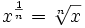| 手机阅读| 用户留言 | 加入收藏 | 设为首页

指数定律_指数定律公式_指数定律方程_指数定律知识指数也叫次方一个数的指数代表把多少个这个数 乘在一起。 在这例子：82 = 8 × 8 = 64 用文字来说：82 也可以叫 "8的2次方"、"8的2次幂" 或 "8的平方"

自己来试试：

11111111111111111111111111111111111

用指数，我们可以少写很多的乘号！

例子：a7

a7 = a × a × a × a × a × a × a = aaaaaaa

留意到我们把英语字母写在一起来代表乘？在这里我们会时常这样写。

例子：x6= xxxxxx

定律的重点
把所有的字母写下来对了解指数定律很重要，例子：x2x3 = (xx)(xxx) = xxxxx = x5，这指出 x2x3 = x5。等会我们会再看这个！所以，如果你有点疑惑，把所有的字母都写下来（数目等于指数），再慢慢仔细看。

所有你需要知道的"指数定律"基于三个理念：指数代表在乘法里用多少次那个数负指数的意思是，因为乘的相反是除分数指数，像 1/n 的意思是取n次方根：若你明白这些，你就明白指数！以下所有的定律都是基于这些理念的。

指数定律
以下为指数定律 （解释在下面）：

x1 = x 61 = 6
x0 = 1 70 = 1
x-1 = 1/x 4-1 = 1/4

xmxn = xm+n x2x3 = x2+3 = x5
xm/xn = xm-n x6/x2 = x6-2 = x4
(xm)n = xmn (x2)3 = x2×3 = x6
(xy)n = xnyn (xy)3 = x3y3
(x/y)n = xn/yn (x/y)2 = x2 / y2
x-n = 1/xn x-3 = 1/x3定律的解释
上面头三个定律（x1 = x, x0 = 1 and x-1 = 1/x）只不过是指数序列的一部分。看：

。。。等等。。。52 1 × 5 × 5 25
51 1 × 5 5
50 1 1
5-1 1 ÷ 5 0.2
5-2 1 ÷ 5 ÷ 5 0.04
。。。等等。。。

留心看列表……留意正指数、零指数和负指数都遵从同一规律，就是大5倍（或小5倍），随着指数增大（或减少）。

xmxn = xm+n 定律

在 xmxn里， 我们把多少个 "x"相乘？答案：先乘"m"次，再乘 "n"次，总共"m+n"次。

xm/xn= xm-n定律

同上，我们把几个 "x"相乘？ 答案："m"次，再减少"n"次（因为是除），总共是"m-n"次。

例子：x4/x2= (xxxx) / (xx) = xx = x2  所以， x4/x2= x(4-2)= x2

（记着 x/x = 1，所以 若在"线上面"有一个x，而在"线下面"也有一个x，你便可以把它们互相消除。）

这个定律也可以显示为什么 x0=1

(xm)n= xmn定律

先乘"m"次，然后重复"n"次，总共m×n次。

例子： (x3)4 = (xxx)4 = (xxx)(xxx)(xxx)(xxx) = xxxxxxxxxxxx = x12  所以 (x3)4 = x3×4 = x12

(xy)n = xnyn 定律

要了解这个定律，想象把所有的"x" 和 "y" 重排，如下：

(x/y)n= xn/yn定律

如上，把 "x" 和 "y" 重排

例子：(x/y)3 = (x/y)(x/y)(x/y) = (xxx)/(yyy) = x3/y3定律

这个定律比较复杂！我建议你先去分数指数看看，否则以下可能会有些混乱，无论如何，重点是：x1/n = x的n次方根

所以一个分数指数，例如 43/2，的意思是以任何次序去取一个立方（3）和一个平方根（1/2）。

记着分数 m/n = m × (1/n)

例子：次序不重要，所以这样 m/n = (1/n) × m 也可以:

例子：好了，就这么多！如果你觉得要记着这么多个定律很困难，不要忘记：

只要你记着及了解，上面的三个理念，你随时可以动动脑筋，自己把定律导出来。

最后，还有一个重要的细节……如果 x= 0 呢？

 正指数（n>0） 0n = 0 负指数（n<0） 未定义！（因为除以 0 是未定义的） 指数 = 0 嗯……看下面！

奇怪的 00

00是什么？有两个说法。

00 可以是 1，或 0，所以有些人说它是"不确定的"：x0 = 1，所以。。。 00 = 1 0n = 0，所以。。。 00 = 0 有疑问…… 00 = "不确定"
更新:20210423 104219

 发表评论

 验证码:看不清楚,点击刷新

.